# Find the volume V of the solid obtained by rotating the region bounded by the given curves about the specified line. 8x...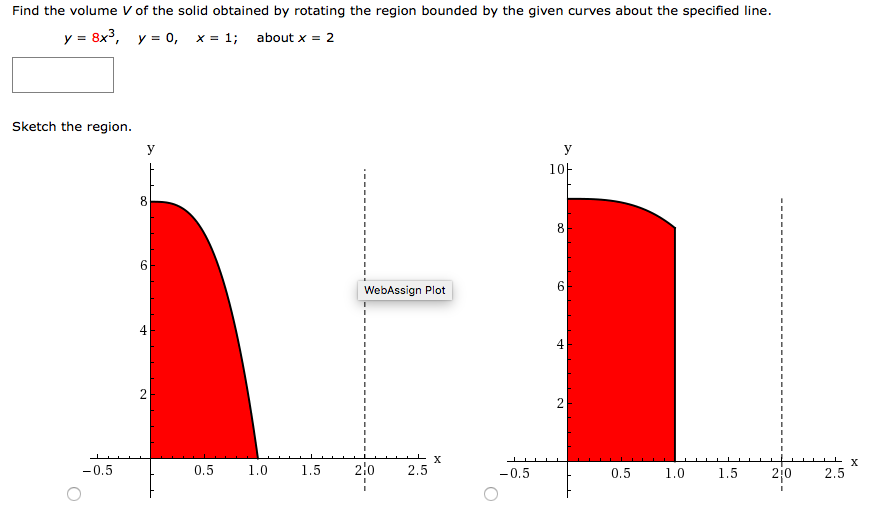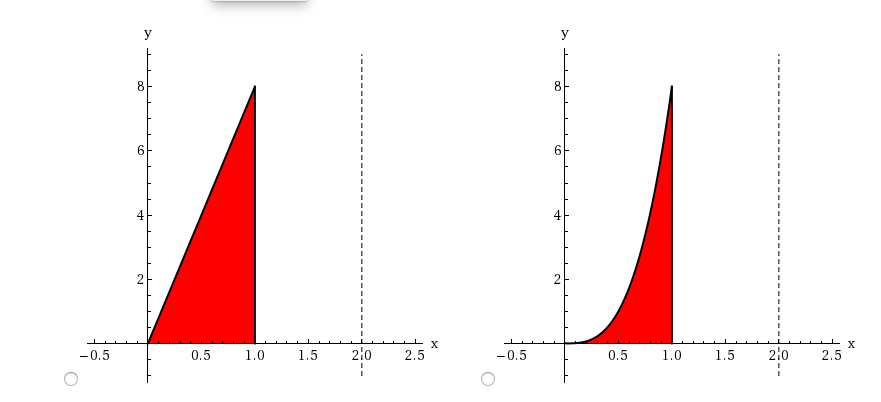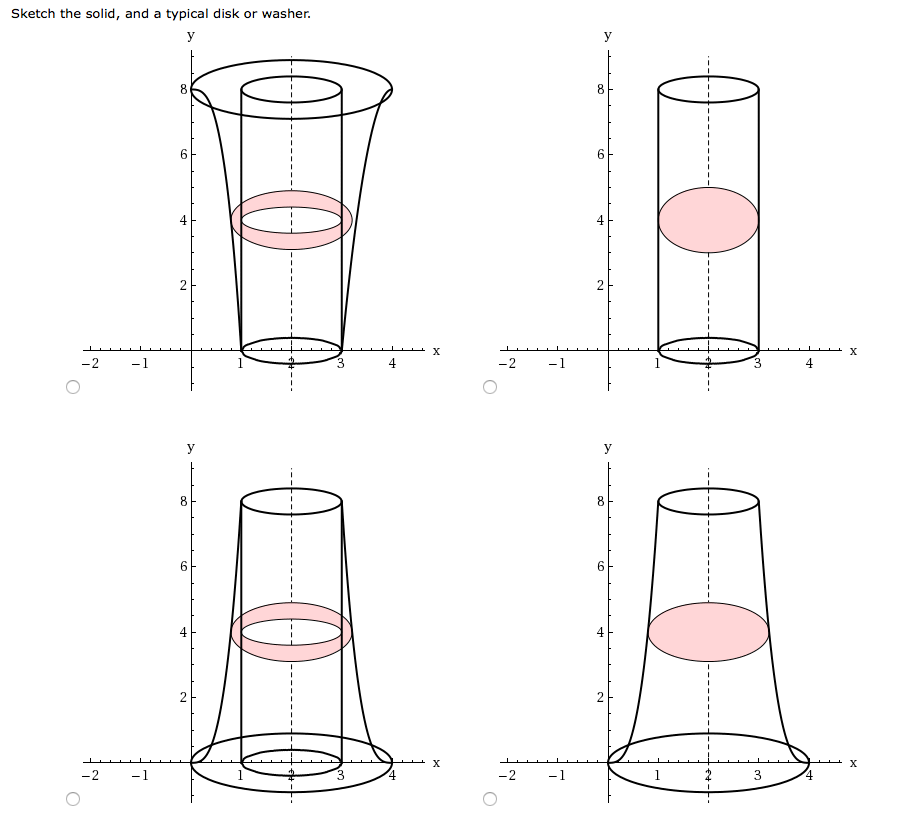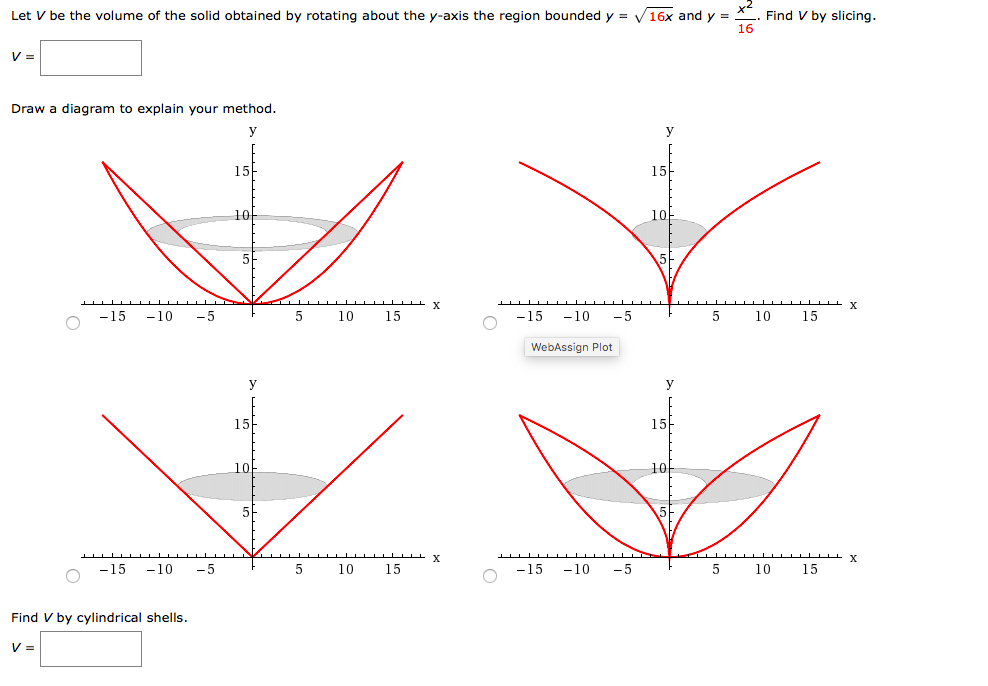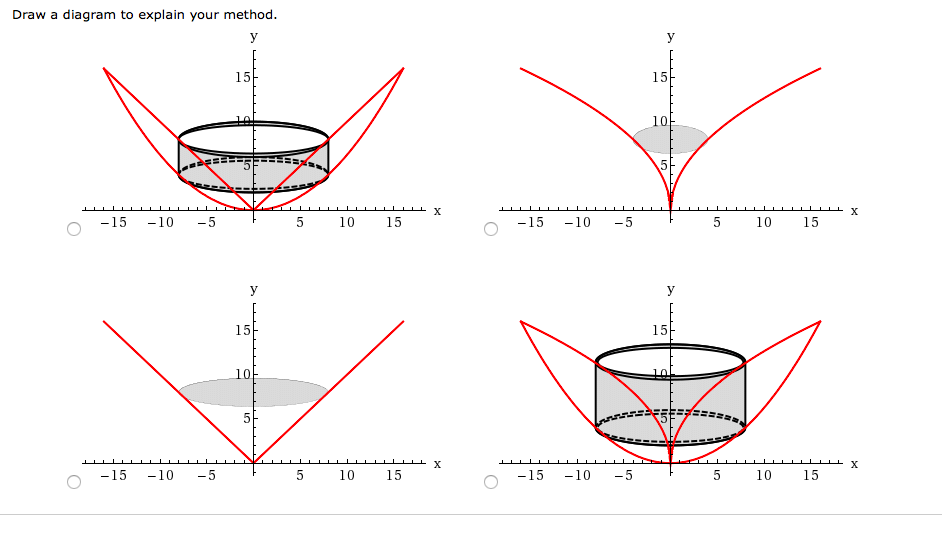Find the volume V of the solid obtained by rotating the region bounded by the given curves about the specified line. 8x3, y 0, x = 1 about x 2 V Sketch the region. 1아 6 WebAssign Plot 4 X 2.5 X 2 0 0.5 0.5 1,0 1,5 2i0 -0.5 0.5 1,0 1.5 2.5 + LC
y 8 4 2 X X 210 210 -0.5 0.5 1,0 1.5 2,5 -0.5 0.5 1,0 1.5 2.5
Sketch the solid, and a typical disk or washer. 8 8 4 2 2 X X 4 -1 -2 4 -2 - 4 2 2 X X 4 3 -1 -2 4 -2
Let V be the volume of the solid obtained by rotating about the y-axis the region bounded y V16x and y = Find V by slicing. 16 V = Draw a diagram to explain your method. 15 15 10 10 X -15 -5 -5 -10 10 15 -15 -10 10 15 WebAssign Plot 15 15 10 10 X -15 -10 -5 -15 -5 10 15 -10 5 10 15 Find V by cylindrical shells. V =
Draw a diagram to explain your method. y 15 15 10 IIL X -15 -10 -5 5 10 15 -15 -10 -5 5 10 15 y 15 15 10 -15 -10 -5 5 10 15 -15 -10 -5 5 10 15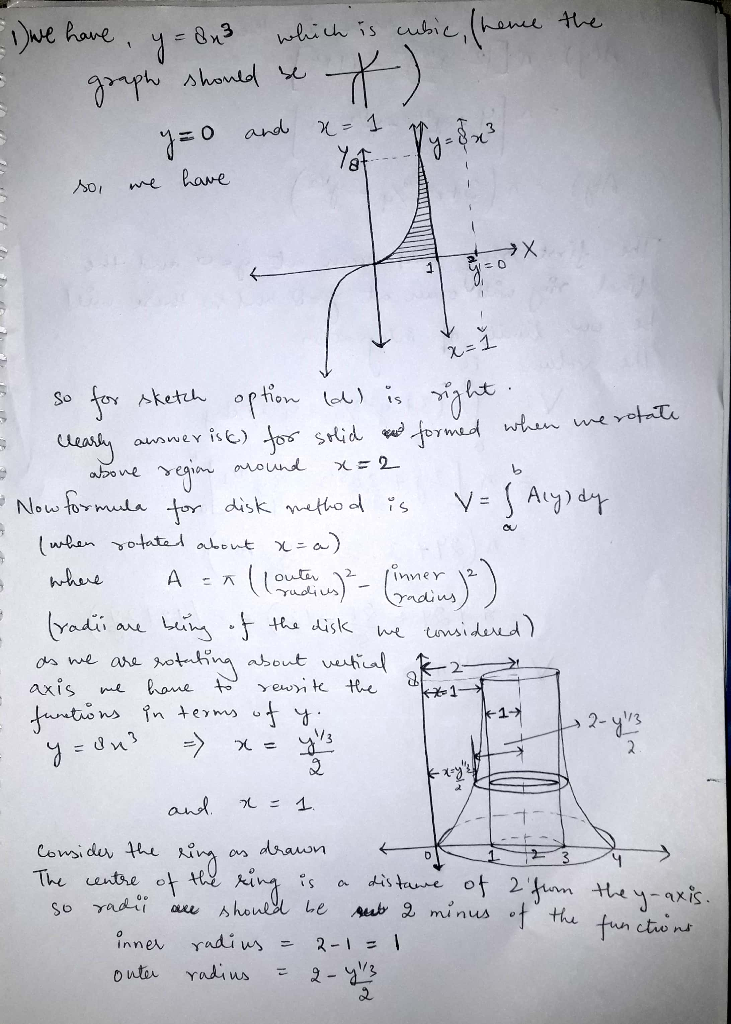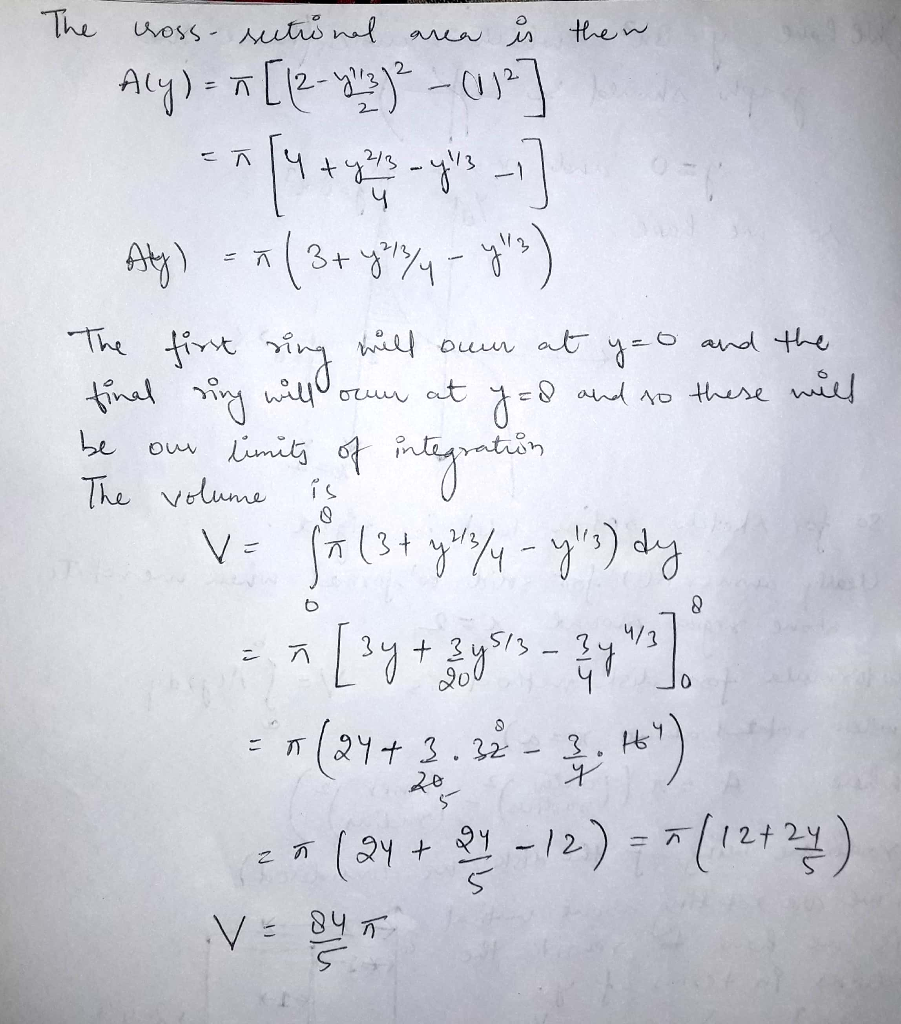##### Add Answer of: Find the volume V of the solid obtained by rotating the region bounded by the given curves about the specified line. 8x...
Similar Homework Help Questions
• ### Find the volume V of the solid obtained by rotating the region bounded by the given curves about the specified line. y-...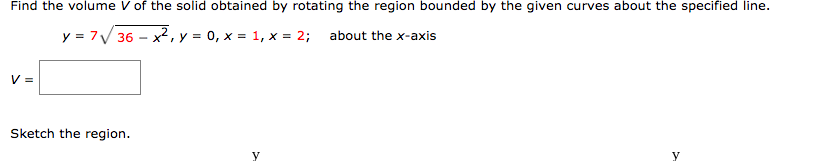Find the volume V of the solid obtained by rotating the region bounded by the given curves about the specified line. y-7V36-,yo,x,x -2; about the x axis Sketch the region Find the volume V of the solid obtained by rotating the region bounded by the given curves about the specified line. y-7V36-,yo,x,x -2; about the x axis Sketch the region

• ### Find the volume V of the solid obtained by rotating the region bounded by the given curves about the specified line. y=...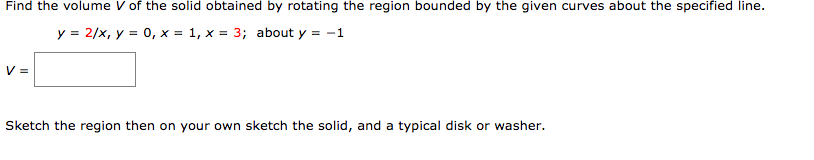Find the volume V of the solid obtained by rotating the region bounded by the given curves about the specified line. y=2/x, y = 0, x=1, x=3; about y =-1 Sketch the region then on your own sketch the solid, and a typical disk or washer. Find the volume V of the solid obtained by rotating the region bounded by the given curves about the specified line. y=2/x, y = 0, x=1, x=3; about y =-1 Sketch the region then...

• ### Find the volume of the solid obtained by rotating the region bounded by the given curves about the specified axis

Find the volume of the solid obtained by rotating the region bounded by the given curves about the specified axis. y=x^(8), y=1 and it is rotated about y=3

• ### 7. Match the volume of the solid obtained by rotating the region bounded by the given curves about about the given axis to the corresponding integra 1, the region bounded by y-V , х--8 and the x-...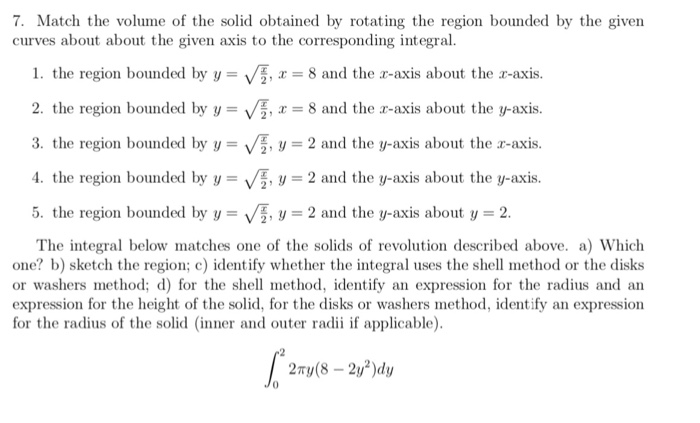7. Match the volume of the solid obtained by rotating the region bounded by the given curves about about the given axis to the corresponding integra 1, the region bounded by y-V , х--8 and the x-axis about the x-axis. 2. the region bounded by 8 and the r-axis about the y-axis. 3, the region bounded by y-V , y-2 and the y-axis about the x-axis. 4. the region bounded by V2 and the y-axis about the y-axis. 5, the...

• ### Find the volume of the solid obtained by rotating the region bounded by the given curves about the specified axis

Find the volume of the solid obtained by rotating the region bounded by the given curves about the specified axis.x=2y^2 , y=1 ,x=0 about the y-axis

• ### Find the volume of the solid obtained by rotating the region bounded by the given curves about the x-axis

Find the volume of the solid obtained by rotating the region bounded by the given curves about the x-axis?y=x^2x=y^2 about the axis x=–2 here's what i did and it was incorrectV = 2pi [x+2][x^1/2 - x^2]dx from 0 to 1V = 2 pi [2x^5/3 / 5 + 4x^3/2 / 3 - x^4/4 - 2x^3 / 3]V = 49/60 :[

• ### find volume of a solid obtained by rotating the region bounded by the given curves about the...

find volume of a solid obtained by rotating the region bounded by the given curves about the specified axisy = x^2 + 1, y = 9 - x^2, about y = -1

• ### Find the Volume of a solid obtained by rotating the region bounded by the given curves about the...

Find the Volume of a solid obtained by rotating the region bounded by the given curves about the specified line. Sketch the region, the solid, and a typical disk orwasher.y = ln x, y = 1, y = 2. x = 0; about the y-axis

• ### Find the volume of the solid obtained by rotating the region bounded by the given curves about the specified axis

Find the volume of the solid obtained by rotating the region bounded by the given curves about the specified axis. y=1/x^2 y=0 x=4 x=7; about y=–3 i integrated and plugged in values for x and got .61 *pi this is not the right answer been trying for hours please help!

• ### Find the volume of the solid obtained by rotating the region bounded by the given curves

A Solid of Revolution. Find the volume of the solid obtained by rotating the region bounded by the given curves about the specified axis.y=5x^2, x=1, y=0, about the x-axis

Need Online Homework Help?